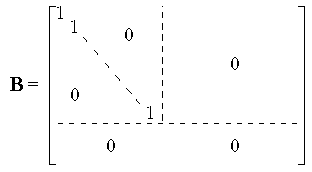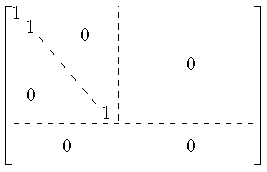# Are linear transformations homomorphism

## Linear maps

Corollary 1 from "Freedom": the coordination map ΦB..

Is B. = {b1, ..., bn} a basis of V, we get one (and only one) linear map

 ΦB. : Kn → V with ΦB.(ei) = b1,
and this is an isomorphism.

The existence of ΦB. follows from the "freedom" of the standard basis of Kn. That ΦB. is an isomorphism follows from criterion 2 for isomorphisms.
 Corollary 1 '. Every vector space of dimension n is isomorphic to K.n.
 Corollary 1 ". All vector spaces of dimension n are isomorphic to one another.
If dim V = dim W = n, then there are isomorphisms ΦV : Kn → V and ΦW. : Kn → W, i.e. the isomorphism ΦW.ΦV-1 : V → W

### Linear maps and matrices

If you limit yourself to finitely generated vector spaces, there is a clear correspondence between linear mappings and matrices - this is thematized in the fields highlighted in light blue:
There is a kind of dictionary of how terms in abstract linear algebra correspond to terms in matrix theory. The fields highlighted in light blue show this.

Every (m × n) matrix A with coefficients in K yields a linear map fA. : Kn → Km, by
 fA.(v) = Av (Matrix multiplication; we understand v as columnsvector on).
is defined.

Are A, B (m × n) matrices with coefficients in K, and is fA. = fB., then A = B.

Conclusion 2 from "Freedom": The reverse is also true!

For every linear mapping f: Kn → Km there is an (m × n) matrix A = M (f) with coefficients in K such that f = fA..

Reformulation:

The assignment that a matrix A in M ​​(m × n, K) is mapped to fA. is a bijection between

and
 the set of linear mappings f: Kn → Km
Note rule for determining the matrix A = M (f).

The linear mappings f: Kn → Km the (m × n) matrix A = M (f) is assigned, with

 the jth column of A is f (ej).

Proof: Here, too, we are dealing with a consequence of "freedom"; again, "freedom" becomes the standard basis of Kn related.
 Composition. fFROM = fA.fB. So: The matrix multiplication (left) corresponds to the series connection of images (right). Invertibility of matrices. Is A in M ​​(m n, K), B in M ​​(n m, K) with AB = Im and BA = In, then m = n.
The invertibility statement follows from the fact that isomorphic vector spaces have the same dimension: Km and Kn are isomorphic only for m = n. (From AB = I and BA = I it follows that fA. and fB. are mutually inverse isomorphisms.)
 Core, image, rankCore (fA.) = Loes (A, 0), Image (fA.) = C (A) (the subspace created by the columns) Rank (fA.) = Rank (A) Then exactly is fA. injective if the columns of A are linearly independent. Then exactly is fA. surjective if the columns of A cover the space Km produce. Then exactly is fA. bijective (i.e. an isomorphism when the columns of A form a basis, i.e. precisely when the matrix A is invertible.

### The interplay of Corollaries 1 and 2:

Let V, W be vector spaces, let dim V = n, dim W = m. Let V a basis of V, W. a basis of V. Then we get a bijection between the linear mappings f: V → W and the (n × m) matrices with coefficients in K.
• A is assigned ΦW. fA.ΦV-1
• f is assigned the matrix representation of ΦW.-1f ΦV.
 fA. Kn → Km ↓ ΦV ↓ ΦW. V → W. f

Is fA. = ΦW.-1 f ΦV, we write A = MVW.(f) and say that A is the performing Matrix of f with respect to the bases V and W. is.

Is f: Kn → Km linear and we denote it with E. each the standard base of Kn as well as from Km, so is ME.E.(f) = M (f).

Is V = {v1, ..., vn} a basis of Kn (understood as a space of column vectors), then let M (V) the matrix whose j-th column is just vj is.
Then: this is the representing matrix of ΦV.

Now let A be an (m × n) matrix with coefficients in K. Consider the map fA.. Base change in Kn and Km delivers:

 fB. Kn → Km ↓ ΦV ↓ ΦW. Kn → Km fA.
So the matrix equation:

Evaluation of the proof of the dimensional formula:

 Sentence. Let V, W be finite-generated vector spaces, let f: V → W be a linear mapping. Then there are bases V from V and W. from W with MVW.(f) = B withLet us apply this theorem to an illustration of the form fA. where A is an (m × n) matrix with coefficients in K, we get a new proof of Theorem 1 "". Compare the two proofs: Our first proof of Theorem 1 "" used elementary row and column operations and is constructive (if the matrix A is given, then one knows exactly how to do to convert A into the given form bring). The new proof, on the other hand, is elegant but not directly constructive: it is based on concepts such as subspace and base, and above all on the supplementary theorem.
Proof: Let f: V → W be linear.
• Be b1, ..., bs a base of kernel (f),
• Continue this with a1, ..., ar to a base of V.
• Then f (a1),...,far) a base of image (f)
(This is precisely what the proof of the dimensional formula for linear mappings says; in particular, r = rank (f).)
• Put f (a1),...,far) by c1, ..., ct on to a base by W.
take V = {a1, ..., ar, b1, ..., bs}
and W. = {f (a1),...,far), c1, ..., ct}.
Then MVW.(f) the specified form.

 Don't be a body. Let A, B (m × n) matrices with coefficients in K. Then the following statements are equivalent: There are vector spaces V, W and a linear map f: V → W such that A and B are representative matrices of f (with respect to any base). fB. is the representational matrix of fA. (on a basis V by Kn and a base W. by Km. There are invertible matrices P, Q with PAQ = B. A, B have the same rank. In this case the matrices are called A and B. equivalent to.. (Mostly one takes the statement (3) as definition of the equivalence of matrices.)
The equivalence of (1), (2) and (3) follows from the considerations on how to assign a matrix to a linear mapping between finite-dimensional vector spaces.

The equivalence between these statements and statement (4) is deeper: If (1) applies, A and B have the same rank, because rank (A) = rank (f) and rank (B) = rank (f), i.e. applies (4).
Conversely, if (4) holds, we have just seen that both fA. as well as fB. have the same representative matrix: